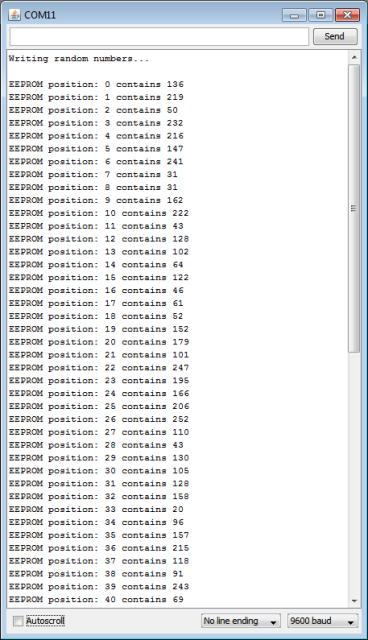# Tutorial: Your Arduino’s inbuilt EEPROM

In this article we are going to examine the internal EEPROM in our Arduino boards. What is an EEPROM some of you may be saying? An EEPROM is an Electrically Erasable Programmable Read-Only Memory.

It is a form of non-volatile memory that can remember things with the power being turned off, or after resetting the Arduino. The beauty of this kind of memory is that we can store data generated within a sketch on a more permanent basis.

Why would you use the internal EEPROM?

For situations where data that is unique to a situation needs a more permanent home. For example, storing the unique serial number and manufacturing date of a commercial Arduino-based project – a function of the sketch could display the serial number on an LCD, or the data could be read by uploading a ‘service sketch’. Or you may need to count certain events and not allow the user to reset them – such as an odometer or operation cycle-counter.

What sort of data can be stored?

Anything that can be represented as bytes of data. One byte of data is made up of eight bits of data. A bit can be either on (value 1) or off (value 0), and are perfect for representing numbers in binary form. In other words, a binary number can only uses zeros and ones to represent a value. Thus binary is also known as “base-2″, as it can only use two digits.

How can a binary number with only the use of two digits represent a larger number? It uses a lot of ones and zeros. Let’s examine a binary number, say 10101010. As this is a base-2 number, each digit represents 2 to the power of x, from x=0 onwards:See how each digit of the binary number can represent a base-10 number. So the binary number above represents 85 in base-10 – the value 85 is the sum of the base-10 values. Another example – 11111111 in binary equals 255 in base 10.Now each digit in that binary number uses one ‘bit’ of memory, and eight bits make a byte. Due to internal limitations of the microcontrollers in our Arduino boards, we can only store 8-bit numbers (one byte) in the EEPROM.

This limits the decimal value of the number to fall between zero and 255. It is then up to you to decide how your data can be represented with that number range. Don’t let that put you off – numbers arranged in the correct way can represent almost anything!

There is one limitation to take heed of – the number of times we can read or write to the EEPROM. According to the manufacturer Atmel, the EEPROM is good for 100,000 read/write cycles (see the data sheet).

One would suspect this to be a conservative estimate, however you should plan accordingly. *Update* After some experimentation, the life proved to be a lot longer

Now we know our bits and and bytes, how many bytes can be store in our Arduino’s microcontroller? The answer varies depending on the model of microcontroller. For example:

• Boards with an Atmel ATmega328, such as Arduino Uno, Uno SMD, Nano, Lilypad, etc. – 1024 bytes (1 kilobyte)
• Boards with an Atmel ATmega1280 or 2560, such as the Arduino Mega series – 4096 bytes (4 kilobytes)
• Boards with an Atmel ATmega168, such as the original Arduino Lilypad, old Nano, Diecimila etc – 512 bytes.

If you are unsure have a look at the Arduino hardware index or ask your board supplier.

If you need more EEPROM storage than what is available with your microcontroller, consider using an external I2C EEPROM as described in the Arduino and I2C tutorial part two.

At this point we now understand what sort of data and how much can be stored in our Arduino’s EEPROM. Now it is time to put this into action. As discussed earlier, there is a finite amount of space for our data. In the following examples, we will use a typical Arduino board with the ATmega328 with 1024 bytes of EEPROM storage.

To use the EEPROM, a library is required, so use the following library in your sketches:

`#include "EEPROM.h"`

The rest is very simple. To store a piece of data, we use the following function:

`EEPROM.write(a,b);`

The parameter a is the position in the EEPROM to store the integer (0~255) of data b. In this example, we have 1024 bytes of memory storage, so the value of a is between 0 and 1023. To retrieve a piece of data is equally as simple, use:

`z = EEPROM.read(a);`

Where z is an integer to store the data from the EEPROM position a. Now to see an example.

This sketch will create random numbers between 0 and 255, store them in the EEPROM, then retrieve and display them on the serial monitor. The variable EEsize is the upper limit of your EEPROM size, so (for example) this would be 1024 for an Arduino Uno, or 4096 for a Mega.

```// Arduino internal EEPROM demonstration

#include <EEPROM.h>
int zz;
int EEsize = 1024; // size in bytes of your board's EEPROM

void setup()
{
Serial.begin(9600);
}
void loop()
{
Serial.println("Writing random numbers...");
for (int i = 0; i < EEsize; i++)
{
zz=random(255);
EEPROM.write(i, zz);
}
Serial.println();
for (int a=0; a<EEsize; a++)
{
Serial.print("EEPROM position: ");
Serial.print(a);
Serial.print(" contains ");
Serial.println(zz);
delay(25);
}
}```

The output from the serial monitor will appear as such:So there you have it, another useful way to store data with our Arduino systems. Although not the most exciting tutorial, it is certainly a useful.

This post brought to you by pmdway.com – everything for makers and electronics enthusiasts, with free delivery worldwide.

To keep up to date with new posts at tronixstuff.com, please subscribe to the mailing list in the box on the right, or follow us on twitter @tronixstuff.